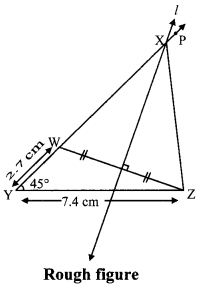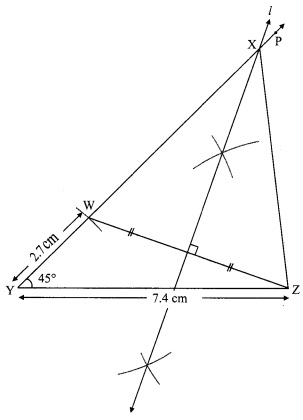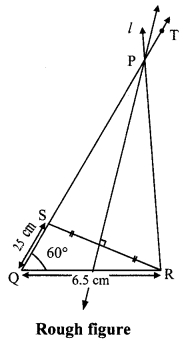# Maharashtra Board Class 9 Maths Solutions Chapter 4 Constructions of Triangles Practice Set 4.2

## Maharashtra State Board Class 9 Maths Solutions Chapter 4 Constructions of Triangles Practice Set 4.2

Question 1.
Construct ∆XYZ, such that YZ = 7.4 cm, ∠XYZ = 45° and XY – XZ = 2.7 cm.
Solution:Here, XY – XZ = 2.7 cm
∴ XY > XZ
As shown in the rough figure draw seg YZ = 7.4 cm
Draw a ray YP making an angle of 45° with YZ
Take a point W on ray YP, such that
YW = 2.7 cm.
Now, XY – XW = YW [Y-W-X]
∴ XY – XW = 2.7 cm ….(i)
Also, XY – XZ = 2.7 cm ….(ii) [Given]
∴ XY – XW = XY – XZ [From (i) and (ii)]
∴ XW = XZ
∴ Point X is on the perpendicular bisector of seg ZW
∴ Point X is the intersection of ray YP and the perpendicular bisector seg ZW

Steps of construction:
i. Draw seg YZ of length 7.4 cm.
ii. Draw ray YP, such that ∠ZYP = 45°.
iii. Mark point W on ray YP such that l(YW) = 2.7 cm.
iv. Join points W and Z.
v. Join the points X and Z.
Hence, ∆XYZ is the required triangle.Question 2.
Construct ∆PQR, such that QR = 6.5 cm, ∠PQR = 60° and PQ – PR = 2.5 cm.
Solution:Here, PQ – PR = 2.5 cm
∴ PQ > PR
As shown in the rough figure draw seg QR = 6.5 cm
Draw a ray QT making on angle of 60° with QR
Take a point S on ray QT, such that QS = 2.5 cm.
Now, PQ – PS = QS [Q-S-T]
∴ PQ – PS = 2.5 cm ……(i) [Given]
Also, PQ – PR = 2.5 cm …..(ii) [From (i) and (ii)]
∴ PQ – PS = PQ – PR
∴ PS = PR
∴ Point P is on the perpendicular bisector of seg RS
∴ Point P is the intersection of ray QT and the perpendicular bisector of seg RS

Steps of construction:
i. Draw seg QR of length 6.5 cm.
ii. Draw ray QT, such that ∠RQT = 600.
iii. Mark point S on ray QT such that l(QS) = 2.5 cm.
iv. Join points S and R.
v. Draw perpendicular bisector of seg SR intersecting ray QT. Name the point as P.
vi. Join the points P and R.
Hence, ∆PQR is the required triangle.Question 3.
Construct ∆ABC, such that BC = 6 cm, ∠ABC = 100° and AC – AB = 2.5 cm.Solution:
Here, AC – AB = 2.5 cm
∴ AC > AB
As shown in the rough figure draw seg BC = 6 cm
Draw a ray BT making an angle of 100° with BC.
Take a point D on opposite ray of BT, :
such that BD 2.5 cm.
Now, AD – AB = BD [A-B-D]
∴ AD – AB = 2.5cm …..(i)
Also, AC – AB = 2.5 cm …..(ii) [Given]
∴ AD – AB = AC – AB [From (i) and (ii)]
∴ Point A is on the perpendicular bisector of seg DC
∴ Point A is the intersection of ray BT and the perpendicular bisector of seg DC

Steps of construction:
i. Draw seg BC of length 6 cm.
ii. Draw ray BT, such that ∠CBT = 100°.
iii. Take point D on opposite ray of BT such that l(BD) = 2.5 cm.
iv. Join the points D and C.
v. Draw the perpendicular bisector of seg DC intersecting ray BT. Name the point as A.
vi. Join the points A and C.
Hence, ∆ABC is the required triangle.